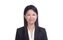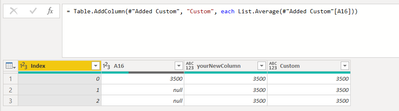cancel
Showing results for
Did you mean:Regular Visitor

## Add column with sepecific cell value

I want create new column A16_2 base on value of first row A16 which use flexible way:

 Index A16 A16_2 0 3500 3500 1 null 3500 2 null 3500

I use Custom function from Edit Query in Excel.

I try some way like that:

1. if [A16] = null then [A16]{0} else [A16]
2. List.Average([A16])

But It NOT WORK. Could someone guide me how to create new column base on specificed value of 1 column?

1 ACCEPTED SOLUTIONSuper User

When you call [A16], it is not a list, so what is your logic?``````let
Source = Table.FromRows(Json.Document(Binary.Decompress(Binary.FromText("i45WMlDSUTI2NTBQitWJVjIEcvJKc3LAHCM4JxYA", BinaryEncoding.Base64), Compression.Deflate)), let _t = ((type nullable text) meta [Serialized.Text = true]) in type table [Index = _t, A16 = _t]),
#"Changed Type" = Table.TransformColumnTypes(Source,{{"Index", Int64.Type}, {"A16", Int64.Type}}),
#"Added Custom" = Table.AddColumn(#"Changed Type", "yourNewColumn", each if [A16]=null then #"Changed Type"[A16]{0} else [A16]),
inSuper User

When you call [A16], it is not a list, so what is your logic?``````let
Source = Table.FromRows(Json.Document(Binary.Decompress(Binary.FromText("i45WMlDSUTI2NTBQitWJVjIEcvJKc3LAHCM4JxYA", BinaryEncoding.Base64), Compression.Deflate)), let _t = ((type nullable text) meta [Serialized.Text = true]) in type table [Index = _t, A16 = _t]),
#"Changed Type" = Table.TransformColumnTypes(Source,{{"Index", Int64.Type}, {"A16", Int64.Type}}),
#"Added Custom" = Table.AddColumn(#"Changed Type", "yourNewColumn", each if [A16]=null then #"Changed Type"[A16]{0} else [A16]),
in